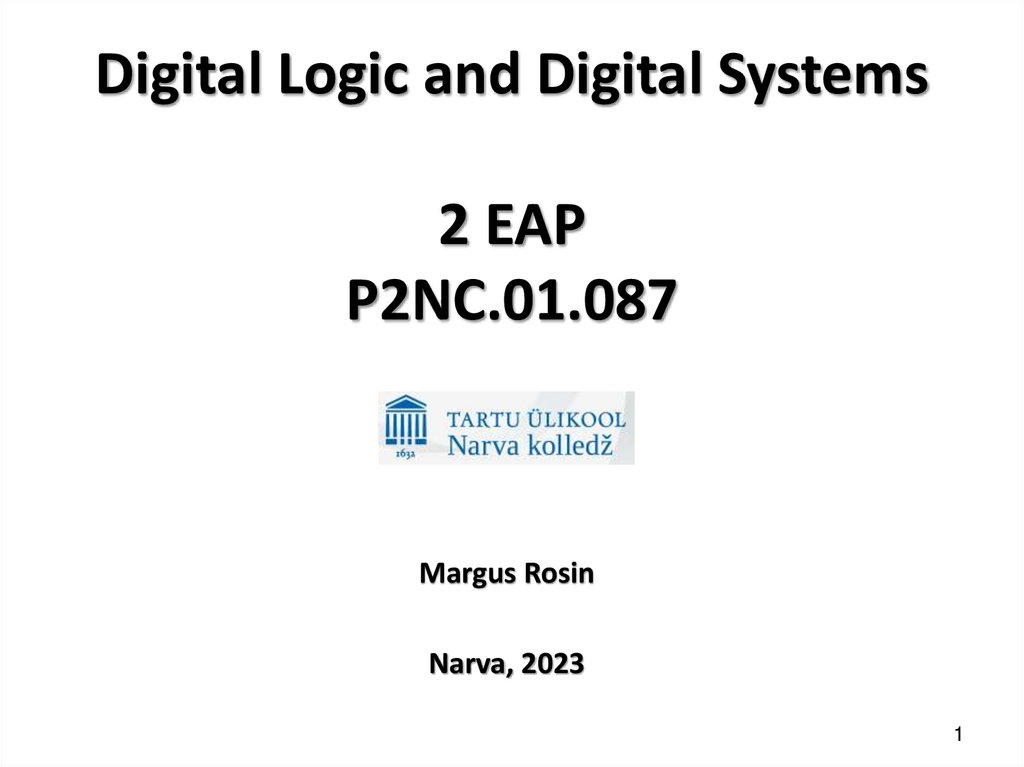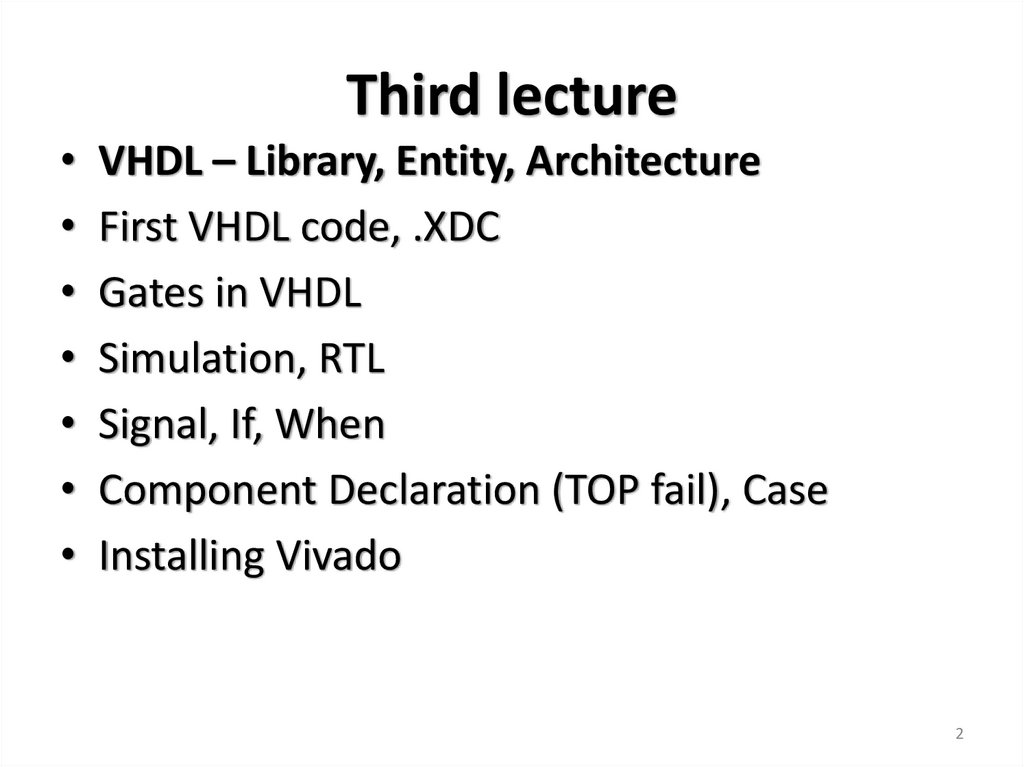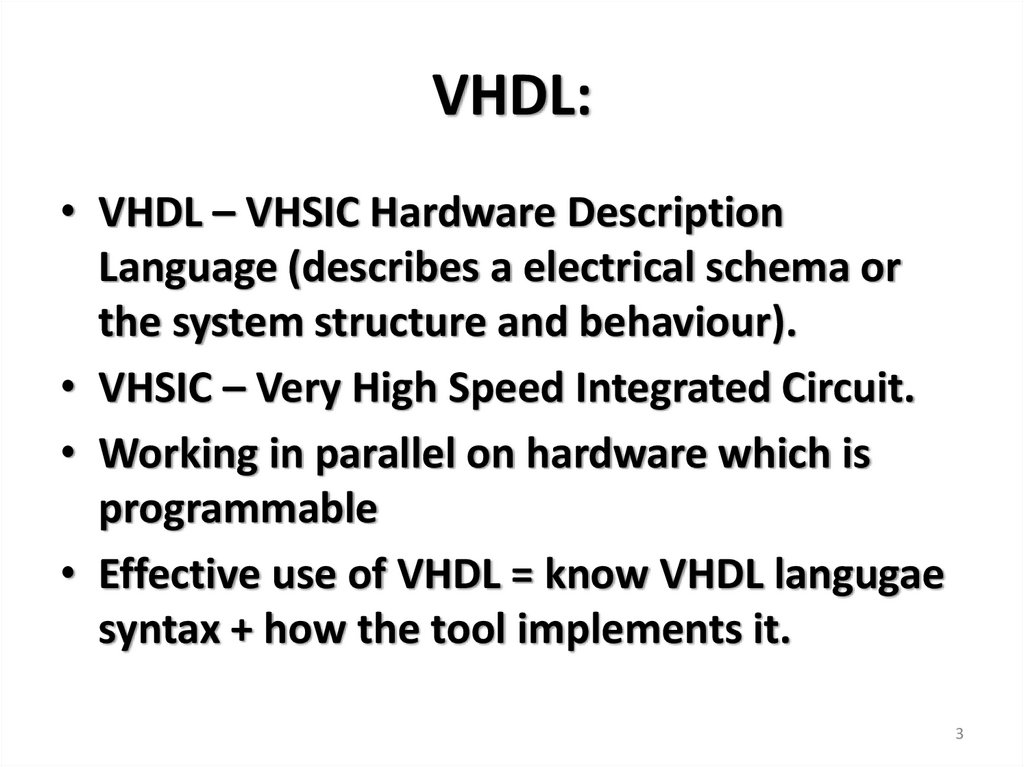# Digital Logic and Digital Systems. Third lecture

Margus Rosin
Narva, 2023
1

## 2. Third lecture

• VHDL – Library, Entity, Architecture
• First VHDL code, .XDC
• Gates in VHDL
• Simulation, RTL
• Signal, If, When
• Component Declaration (TOP fail), Case
2

## 3. VHDL:

• VHDL – VHSIC Hardware Description
Language (describes a electrical schema or
the system structure and behaviour).
• VHSIC – Very High Speed Integrated Circuit.
• Working in parallel on hardware which is
programmable
• Effective use of VHDL = know VHDL langugae
syntax + how the tool implements it.
3

## 4. VHDL IEEE

• Out of the United States Department of Defence program
what gave the rights over to IEEE
• 1987 – first VHDL standard IEEE 1076-1987
• 1994 – updated standard – 1076-1993 – VHDL `93
• 2000 – protected types, variable – 1076-1993 – VHDL 2000
• 2002 – 1076-2000 – VHDL 2002
• 2008 – external names etc.
• VHDL-AMS (Analog and Mixed Signal Extensions for VHDL)
4

## 5. FPGA programming:

• 1) VHDL code – design and test
(testbench).
– 1a) Not to technology related
optimization and minimization.
– 1b) Simulation.
• 2) Netlist
• 3) Mapping to choosen
technology + place + router
• 4) Bitstream to FPGA
5

## 6. Tools

• Front-end:
– Design collector - text editor, visual.
– Simulator – testbench (no netlist needed).
– Synthesize – producing the netlist.
• Back-end:
– Mapping (the netlist) – to the given technology
– Place ja Route – setting the exact slices
– (Statical) timing anaylses –longest path in less then 1 clock cycle.
– Hardware – bitstream to FPGA.
6

## 7. VHDL - .vhd

• Synthesizable (inc. simulation) and only
simulation.
• Project name is: .xpr (Xilinx ISE had xise). Project
has several subfolders containing a lot of files.
• Design itself is .vhd (same name as ENTITY)
• I/O defined in Constraints file .xdc (ISE had .ucf)
(comment is “#”):
set_property -dict {PACKAGE_PIN V17 IOSTANDARD LVCMOS33} [get_ports a];
set_property -dict {PACKAGE_PIN E19 IOSTANDARD LVCMOS33} [get_ports {f}];
• Bitstream to FPGA is .bit (PROM also .bit))
7

## 8. VHDL code 3 components

Library (defines variables behaviour, usable
components etc)
Entity (defines I/O)
Architecture (defines
functionality)
8

9

## 10. Library

• Mostly needed are added automatically, some
are just commented out.
-- This row is a comment, begins with two “-”
-- comment...
library IEEE;
use IEEE.STD_LOGIC_1164.ALL;
10

## 11. Block comment

• One line: -• To comment multiple rows either:
• Right-click -> Toggle Line Comments
• Ctrl + Slash
11

## 12. Mostly needed libraries:

library IEEE;
use IEEE.numeric_std.all; -- need to uncomment
use IEEE.std_logic_unsigned.all;
12

## 13. Less needed:

use IEEE.math_complex.all;
use IEEE.math_real.all;
use IEEE.numeric_bit.all;
use IEEE.std_logic_misc.all;
use IEEE.std_logic_signed.all;
use std.textio.all;
13

## 14. Entity:

entity <entity_name> is
[ generic (
<generic_name> : <type> := <value>;
<other generics>...
); ] -- between [ ] is a might be part, not has to be
port (
<port_name> : <mode> <type>;
<other ports>...
);
end <entity_name>;
14

## 15. Entity example:

ENTITY xor_v2rat IS
PORT (a, b : IN BIT;
x : OUT BIT);
END xor_v2rat;
15

## 16. Architecture:

architecture <arch_name> of <entity_name> is
[ -- declarative_items (signal declarations,
-- component declarations, etc.) ]
begin
-- architecture body
end <arch_name>;
16

## 17. Architecture example

ARCHITECTURE xor_v2rat_A OF xor_v2rat IS
BEGIN
x <= a XOR b; -- “<=” – firstly we do the
rightmost part of <= and then assigning the
value to x.
END xor_v2rat_A;
17

## 18. Entity reserved names

• ENTITY and ARCHITECTURE name can be
whatever except reserved names:
More
18
https://www.xilinx.com/support/documentation/sw_manuals/xilinx11/ite_r_vhdl_reserved_words.htm

## 19. Third lecture

• VHDL – Library, Entity, Architecture
• First VHDL code, .XDC
• Gates in VHDL
• Simulation, RTL
• Signal, If, When
• Component Declaration (TOP fail), Case
19

20

## 21. First VHDL code

ENTITY ex1 IS
PORT (x1, x2, x3 : IN BIT;
f : OUT BIT);
END ex1;
ARCHITECTURE ex1_arh OF ex1 IS
BEGIN
f <= (x1 AND x2) OR NOT(NOT x2 AND x3);
END ex1_arh;
NB! A signal name has to begin with a letter and can’t be a VHDL reserved name!
21

## 22. Parentheses!

• VHDL supports AND, OR, NOT, NAND, NOR, XOR ja
XNOR.
• Parentheses! x2 OR NOT x2 AND x3 would produce an
error:
[Synth 8-2715] syntax error near or ["...first_A.vhd":47]
Correct would be: x2 OR (NOT x2 AND x3) | x2 OR NOT
(x2 AND x3 ) – PS! Different result!
Also correct would be: NOT x1 OR x2 OR NOT x3
F <= (x1 AND x2) OR NOT(NOT x2 AND x3);
22

## 23. Third lecture

• VHDL – Library, Entity, Architecture
• First VHDL code, .XDC
• Gates in VHDL
• Simulation, RTL
• Signal, If, When
• Component Declaration (TOP fail), Case
23

## 24. Gates in VHDL

library IEEE;
use IEEE.STD_LOGIC_1164.ALL;
Entity Gate_1 is
port( a, b : in STD_LOGIC;
z : out STD_LOGIC_VECTOR(5 downto 0) );
end Gate_1;
Architecture Gate_1_arch of Gate_1 is
Begin
z(5) <= a and b;
More then 1 input:
z(4) <= a nand b;
Let’s have Input (0 to n) and
z(3) <= a or b;
Output <= Input(0) <OPERATION> Input(1)
z(2) <= a nor b;
<OPERATION> .. <OPERATION> Input(n)
z(1) <= a xor b;
AND – output is a 1 if all inputs are 1.
z(0) <= a xnor b;
OR – OUT 0 if all IN are 0.
End Gate_1_arch;
NAND – OUT 0 if all IN are 1
Gates in VHDL
NOR – OUT 1 if all IN are 0.
XOR and XNOR ???
XOR – OUT 1 if IN that have 1 are odd.
XNOR – OUT 1 if IN that have 1 are even.
24

## 25. Testing NAND4 ja XOR4

testbench : PROCESS
BEGIN
a <= '0'; b <= '0';
c <= '0'; d <= '0';
wait for 10ns;
a <= '1';
wait for 10ns;
b <= '1';
wait for 10ns;
c <= '1';
wait for 10ns;
d <= '1';
WAIT; -- waits forever
END PROCESS;
25

## 26. More than 2 inputs:

Input f and output z are STD_LOGIC_VECTOR(5 downto 0)
z(5) <= f(5) and f(4) and f(3) and f(2) and f(1) and f(0);
z(4) <= f(5) nand f(4);
z(3) <= f(5) or f(4) or f(3) or f(2) or f(1) or f(0);
z(2) <= f(5) nor f(4);
z(1) <= f(5) xor f(4) xor f(3) xor f(2) xor f(1) xor f(0);
z(0) <= f(5) xnor f(4) xnor f(3) xnor f(2) xnor f(1) xnor f(0);
Why nand and nor have only 2 inputs at a time?
26

## 27. NAND and NOR speciality:

Vivado doesn’t support. (Syntax error near
"nand". Also Xilinx ISE didn’t support)
Parentheses help out:
z(4) <= ((f(5) nand f(4)) nand (f(3) nand f(2)))
nand (f(1) nand f(0)); -- works.
z(4) <= (f(5) nand f(4)) nand (f(3) nand f(2))
nand (f(1) nand f(0)); -- why this isn’t?
27

## 28. NOR vs NOR

...
Port ( In1 : in STD_LOGIC_VECTOR (0 to 3);
Out1, Out2 : out STD_LOGIC);
...
Out1 <= ((in1(0) nor in1(1)) nor in1(2)) nor in1(3);
Out2 <= (in1(0) nor in1(1)) nor (in1(2) nor in1(3));
...
28

## 29. Third lecture

• VHDL – Library, Entity, Architecture
• First VHDL code, .XDC
• Gates in VHDL
• Simulation, RTL
• Signal, If, When
• Component Declaration (TOP fail), Case
29

30

## 31. Test Bench

LIBRARY ieee; USE ieee.std_logic_1164.ALL; USE ieee.numeric_std.ALL;
ENTITY testbench IS
END testbench;
Test Bench
ARCHITECTURE behavior OF testbench IS
COMPONENT <component name> -- Component Declaration
PORT(<port1> : IN std_logic_vector(3 downto 0); -- whta signal we want to use in simulation
<port2> : OUT std_logic_vector(3 downto 0));
END COMPONENT;
SIGNAL <signal1> : std_logic_vector(3 downto 0); -- simulation signals
SIGNAL <signal2> : std_logic_vector(3 downto 0);
BEGIN
uut: <component name> PORT MAP( -- Component Instantiation. UUU - UnitUnderTest
<port1> => <signal1>,
<port2> => <signal2>);
tb : PROCESS -- Test Bench Statements
BEGIN
wait for 100 ns;
-- Add user defined stimulus here with signals
wait; -- will wait forever
END PROCESS tb; -- End Test Bench
31
END;

## 32. Simulation

signal a, b : std_logic := '0';
stim_proc: process
begin
for i in 0 to 1 loop
a <= not a;
for j in 0 to 1 loop
b <= not b;
wait for 10ns;
end loop;
end loop;
wait;
end process;
Input a and b pass the gates: and, nand, or,
nor, xor ja xnor
32

## 33. 4IN-2OUT – find 4 error in the code!

ENTITY n2ide2 IS
PORT (x1, x2, x3 : IN BIT;
f, g : OUT BIT);
END n2ide2;
ARCHITECTURE n2ide2 OF n2ide3
IS
BEGIN
f <= (x1 AND x3) OR (x2 AND
x4);
g IS (x1 OR NOT x3) AND (NOT
x2 OR x4)
END n2ide2;
33

## 34. Cin and Cout VHDL code:

library IEEE;
use IEEE.STD_LOGIC_1164.ALL;
entity Skeem_1 is
port ( a : in std_logic; -- can be written as: a, b, cin: in
b : in std_logic;
cin : in std_logic;
cout : out std_logic);
end Skeem_1;
architecture Skeem_1_A of Skeem_1 is
begin
cout <= (a AND b) OR (a AND cin) OR (b AND cin);
end Skeem_1_A;
34

## 35. Previous RTL:

cout <= (a AND b) OR (a AND cin) OR (b AND cin);
35

## 36. Implementation in CPLD and FPGA

• f = !x1!x2!x3+!x1x2!x3+x1!x2!x3+x1!x2x3+x1x2!x3
CPLD
FPGA
36

## 37. Write VHDL code that implements

• f(x1,x2,x3) = SUM of m(0,3,6).
x1
0
x2
0
x3
0
f
1
0
0
0
0
1
1
1
0
1
0
0
1
1
1
1
0
0
1
0
1
0
0
0
1
1
1
1
0
37

## 38. 3 IN & 1 OUT –> f=!x3+x1!x2

3 IN & 1 OUT –> f=!x3+x1!x2
library IEEE;
use IEEE.STD_LOGIC_1164.ALL;
entity in4 is
Port ( x1, x2, x3: in STD_LOGIC;
f : out STD_LOGIC);
end in4;
architecture arc4 of in4 is
begin
f <= (NOT x1 AND NOT x2 AND NOT x3) OR (NOT x1 AND x2 AND NOT x3) OR
(x1 AND x2 AND NOT x3) OR (x1 AND NOT x2 AND NOT x3) OR (x1 AND NOT x2
AND x3);
end arc4;
38

## 39. RTL and after Synthesis

39

O=!I0 + I1 & !I2
I1 == x1 , I2 == x2,
I0 == x3, G == f.
So f = !x3+x1!x2
40

## 41. Third lecture

• VHDL – Library, Entity, Architecture
• First VHDL code, .XDC
• Gates in VHDL
• Simulation, RTL
• Signal, If, When
• Component Declaration (TOP fail), Case
41

## 42. Entity signaal

• A signal can works as: IN, OUT, INOUT xor
BUFFER:
• Signal types: BIT, STD_LOGIC, INTEGER etc.
42

## 43. 2-to-1 MUX

• y = a if s = 0 and y = b if s = 1
...
a, b, s : in STD_LOGIC;
y : out STD_LOGIC;
...
y <= (not s and a) or (s and b);
...
ab(to right)
s (down)
00
01
11
10
0
0
0
1
1
1
0
1
1
0
y = !s & a | s & b
43

## 44. If:

[Name: ] if <condition> then
<statement> -- usually each statement will be
synthesized as a mux
elsif <condition> then -- you can have n amount of
elsif.
<statement>
else -- if we don’t describe all possible cases we
might end up with a latch.
<statement>
[null; -- does nothing]
end if;
44

## 45. If example

if C1 = '1' and C2 = '1' then
V := not V;
W := '0';
elsif C4 = '0' then
X := B;
else X := C;
end if;
45

## 46. If example

Mux: process (Sel, A, B)
begin
if Sel = '0' then -- synthesize gives a mux
F <= A;
else
F <= B;
end if;
end process;
Latch: process (En, D)
begin
if En = '1' then
Q <= D; -- not complete assign -> latch
end if;
end process;
46

## 47. If example

Dtype: process
begin
wait until Clock = '1';
if En = '1' then
Q <= D; -- flipflop synthesized
end if;
end process;
DtypeR: process (Reset, Clock)
begin
if Reset = '0' then -- synthesizes gives an asynchronous reset
Q <= '0';
elsif Clock'Event and Clock = '1' then
Q <= D; -- flipflop
end if;
end process;
47

## 48. When / Else

<name> <= <expression> when <condition> else
<expression> when <condition> else
<expression>;
48

## 49. Third lecture

• VHDL – Library, Entity, Architecture
• First VHDL code, .XDC
• Gates in VHDL
• Simulation, RTL
• Signal, If, When
• Component Declaration (TOP fail), Case
49

## 50. Using several .vhd files in one project

• When we use multiple files we get an
opportunity to call the different files with
described functionalities multiple times.
• For example let’s have 2 files out of one is a
TOP file and the second describes some
functionality. On the TOP file architecture we
write component declaration (what ports we
want to use from the functional file) and port
mapping.
50

## 51. Component Declaration:

component <component_name>
[ generic (
<generic_name> : <type> := <value>;
<other generics>...
); ]
port (
<port_name>[, <port_name_2>] : <mode> <type>;
<other ports>...
);
end component;
51

## 52. Component Instantiation:

<instance_name> : <component_name>
[ generic map (
<generic_name> => <value>,
<other generics>... ) ]
port map ( -- name association
<port_name> => <signal_name>,
[ UpDown => open, -- unconnected]
<other ports>...
);
[ port map (Clk64Mhz, RST, open, Count); -position association ]
52

## 53. TOP file:

library IEEE;
use IEEE.STD_LOGIC_1164.ALL;
entity V2ratid_TOP is
Port ( liugnupp : in STD_LOGIC_VECTOR (1 downto 0);
leed : out STD_LOGIC_VECTOR (5 downto 0));
end V2ratid_TOP;
TOP file:
architecture V2ratid_TOP of V2ratid_TOP is
component V2ratid_kood is
port(
a : in STD_LOGIC;
b : in STD_LOGIC;
z : out STD_LOGIC_VECTOR(5 downto 0));
end component;
As both arrays have the same lenght
begin
we don’t need to write them separately
Suvanimi : V2ratid_kood port map(
a => liugnupp(1),
b => liugnupp(0),
z => leed
);
end V2ratid_TOP;
53

54

## 55. 3 subfiles and 1 TOP

• Each subfiles realizes on different way a 2-to-1
MUXi.
• Topfile works as 4-to-1 MUX
55

## 56. TOP_Mux41.vhd

...
entity TOP_Mux41 is
Port ( c : in STD_LOGIC_VECTOR (3 downto 0);
s : in STD_LOGIC_VECTOR (1 downto 0);
z : out STD_LOGIC);
end TOP_Mux41;
architecture mux41 of TOP_Mux41 is
component mux_21 is
port(a1, b1, s1 : in std_logic; y1 : out std_logic);
end component;
component mux_21_when is
port(a2, b2, s2 : in std_logic; y2 : out std_logic);
end component;
component mux_21_if is
port(a3, b3, s3 : in std_logic; y3 : out std_logic);
end component;
signal v, w : STD_LOGIC; -- BEFORE begin, architecture internal signals!
begin
M1: mux_21 port map
(a1 => c(0), b1 => c(1), s1 => s(0), y1 => v);
M2: mux_21_when port map
(a2 => c(2), b2 => c(3), s2 => s(0), y2 => w);
M3: mux_21_if port map
(a3 => v, b3 => w, s3 => s(1), y3 => z);
end mux41;
TOP_Mux41.vhd
56

## 57. 3 x 2-to-1 MUX

entity mux_21 is
Port ( a1, b1, s1 : in STD_LOGIC;
y1 : out STD_LOGIC);
end mux_21;
architecture mux_21 of mux_21 is
begin
y1 <= (not s1 and a1) or (s1 and b1);
end mux_21;
entity mux_21_when is
Port ( a2, b2, s2 : in STD_LOGIC;
y2 : out STD_LOGIC);
end mux_21_when;
architecture mux_21_when of mux_21_when is
begin
y2 <= a2 when s2 = '0' else b2;
3 x 2-to-1 MUX
entity mux_21_if is
Port ( a3, b3, s3 : in STD_LOGIC;
y3 : out STD_LOGIC);
end mux_21_if;
architecture mux_21_if of mux_21_if is
begin
protsess1 : process (a3, b3, s3)
begin
if s3 = '0' then y3 <= a3;
else y3 <= b3;
end if;
end process;
end mux_21_if;
end mux_21_when;
57

## 58. TestBench

s(0) <= '0';
s(1) <= '0';
for j in 0 to 3 loop
c(0) <= NOT c(0);
for i in 0 to 3 loop
c(1) <= NOT c(1);
for ii in 0 to 3 loop
c(2) <= NOT c(2);
for jj in 0 to 3 loop
c(3) <= NOT c(3);
wait for 5ns;
end loop;
end loop;
end loop;
end loop;
wait for 10 ns;
58

## 59. 4-to-1 MUX simpler

Entity mux41 is
port (c : in STD_LOGIC_VECTOR(3 downto 0);
s : in STD_LOGIC_VECTOR(1 downto 0);
z : out STD_LOGIC);
end mux41;
Architecture mux41a of mux41 is
Begin
Z <= (not s(1) and not s(0) and c(0))
or (not s(1) and
s(0) and c(1))
or (
s(1) and not s(0) and c(2))
or (
s(1) and
s(0) and c(3));
End mux41a;
59

## 60. Case

[Nimetus: ] case (<2-bit select>) is -- 1 to n bit.
when "00" =>
<statement>;
when "01" =>
<statement>;
when "10" | "11" => -- 10 OR 11. Can be also: when 2 to 15.
<statement>;
when others =>
<statement>;
end case;
60

## 61. 4-to-1 MUX

Architecture...
Begin
Protsess1 : process (c, s)
Begin
case s is
when ”00” => z <= c(0);
when ”01” => z <= c(1);
when ”10” => z <= c(2);
when ”11” => z <= c(3);
when others => z <= c(0);
end case;
End process;
...
61

## 62. Third lecture

• VHDL – Library, Entity, Architecture
• First VHDL code, .XDC
• Gates in VHDL
• Simulation, RTL
• Signal, If, When
• Component Declaration (TOP fail), Case
62

63

## 64. Vivado HLx WebPACK install

• Xilinx Unified Installer 2022.2: Windows Self Extracting Web
Installer (EXE - 209.61 MB)
- oma ülikooli andmed).
• Unified Xilinx Installer aken võib paar minutit võtta - oota
rahulikult.
• Xilinx Unified 2022.2 Installer aknas Next -> User Auth. ja
Vivado ML Standard ja Next ->
• Devices alt jäta alles ainult 7 Series all olev Artix-7 ja Next ->
3 x Agree ja Next -> Install
64

65